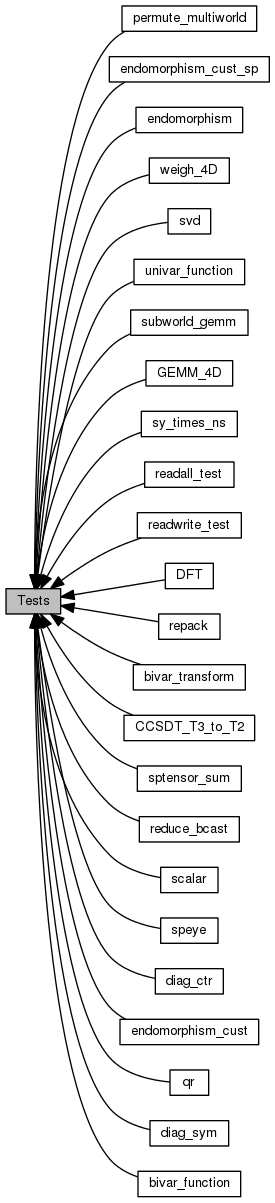Cyclops Tensor Framework parallel arithmetic on multidimensional arrays
Tests

Matrix multiplication. More...

Collaboration diagram for Tests:## Modules

qr
QR factorization of CTF matrices.

svd
SVD factorization of CTF matrices.

bivar_function
tests custom element-wise functions by implementing division elementwise on 4D tensors

bivar_transform
tests custom element-wise transforms by implementing division elementwise on 4D tensors

DFT
Discrete Fourier Transform by matrix multiplication.

diag_ctr
Summation along tensor diagonals.

diag_sym
Summation along tensor diagonals.

endomorphism
tests custom element-wise functions by implementing division elementwise on 4D tensors

endomorphism_cust
tests custom element-wise functions by implementing division elementwise on 4D tensors

endomorphism_cust_sp
tests cust_spom element-wise functions by implementing division elementwise on 4D tensors

GEMM_4D
Folded matrix multiplication on 4D tensors.

permute_multiworld
tests permute function between different worlds

Summation along tensor diagonals.

Tests how writes to diagonals are handled for various tensors.

reduce_bcast
Summation along tensor diagonals.

repack
Tests contraction of a symmetric index group with a nonsymmetric one.

scalar
Basic functionality test for CTF::Scalar<> type and tensors with a zero edge length.

speye
Sparse identity matrix test.

sptensor_sum
Summation of sparse tensors.

subworld_gemm
Performs recursive parallel matrix multiplication using the slice interface to extract blocks.

sy_times_ns
Tests contraction of a symmetric index group with a nonsymmetric one.

univar_function
tests custom element-wise functions by implementing division elementwise on 4D tensors

weigh_4D
tests custom element-wise functions by implementing division elementwise on 4D tensors

CCSDT_T3_to_T2
A symmetric contraction from CCSDT compared with the explicitly permuted nonsymmetric form.

## Functions

int multi_tsr_sym (int m, int n, World &dw)

char * getCmdOption (char **begin, char **end, const std::string &option)

int main (int argc, char **argv)

## Detailed Description

Matrix multiplication.

## Function Documentation

 char* getCmdOption ( char ** begin, char ** end, const std::string & option )

Definition at line 62 of file multi_tsr_sym.cxx.

Referenced by main().

 int main ( int argc, char ** argv )

Definition at line 72 of file multi_tsr_sym.cxx.

References getCmdOption(), multi_tsr_sym(), ctf.core::np(), and ctf.core::rank().

 int multi_tsr_sym ( int m, int n, World & dw )

Definition at line 13 of file multi_tsr_sym.cxx.

Referenced by main().